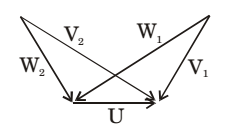## Fluid Mechanics and Hydraulic Machinery Miscellaneous

#### Fluid Mechanics and Hydraulic Machinery

1. For a Newtonian fluid

1. For a Newtonian fluid, shear stress is proportional to rate of shear strain.

##### Correct Option: C

For a Newtonian fluid, shear stress is proportional to rate of shear strain.

1. Kaplan water turbine is commonly used when the flow through its runner is

1. Kaplan turbine is an axial flow turbine and works at low heads (<50).

##### Correct Option: B

Kaplan turbine is an axial flow turbine and works at low heads (<50).

1. In order to have maximum power from a Pelton turbine, the bucket speed must be

1. For a pelton wheel, maximum power is obtained at,

 u = υ 2

##### Correct Option: B

For a pelton wheel, maximum power is obtained at,

 u = υ 2

1. The velocity triangles at the inlet and exit of the rotor of a turbo machine are shown. V denotes the absolute velocity of the fluid, W denotes the relative velocity of the fluid and U denotes the blade velocity. Subscripts 1 and 2 refer to inlet and outlet respectively If V2 = W1 and V1 = W2, then the degree of reaction is1.  DOR = W2² −W1² 2/W2² −W1²

 = 1 = 0.5 2

##### Correct Option: C

 DOR = W2² −W1² 2/W2² −W1²

 = 1 = 0.5 2

1. A hydraulic turbine develops 1000 kW power for a head of 40 m. If the head is reduced to 20 m, the power developed (in kW) is

1.  Pu = P H3/2

 ∴ P1 = P2 H13/2 H23/2

⇒  P2 = 353.55 kW.

##### Correct Option: B

 Pu = P H3/2

 ∴ P1 = P2 H13/2 H23/2

⇒  P2 = 353.55 kW.